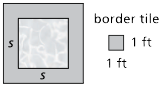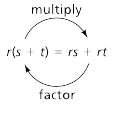Toggle Accessibility Tools

# 8-6 Say It With Symbols - Concepts and Explanations

## Equivalent Expressions

In previous Units, students explored ways in which relationships can be expressed in tables, graphs, and equations. Often, the contextual clues or the patterns in tables or graphs could only be represented by one form of an equation. Here, students are deliberately presented with situations in which contextual clues can be interpreted in several ways to produce different but equivalent equations

### Example

Find the number of 1-foot-square tiles N needed to make a border around a square pool with sides of length s feet.

Different conceptualizations of the situation can lead to different but equivalent expressions for the number of tiles:N = 4s +4
N = 4(s + 1);
N = s + s + s + s + 4
N = 8 + 4(s - 1)
N = 2s + 2(s + 2)
N = (s + 2)2 - s2

## Revisiting the Distributive Property

If an expression is written as a factor multiplied by a sum of two or more terms, the Distributive Property can be applied to multiply the factor by each term in the sum. If an expression is written as a sum of terms and the terms have a common factor, the Distributive Property can be applied to rewrite the expression as the common factor multiplied by a sum of two or more terms. This process is called factoring.

### ExampleThe Distributive Property allows you to group symbols (shown on the left side of the equation) or to expand an expression as needed (shown on the right side of the equation)

## Checking for Equivalence

Students may use geometric reasoning to decide if expressions are equivalent. Students may check whether equivalent. Students may check whether equations have the same graphs and tables. Students should also be able to use the Distributive and Commutative properties to show that expressions are equivalent.

### Example

By applying the Distributive Property, 4(s + 1) = 4s + 4.
8 + 4(s - 1) can be shown to be equivalent to 4s + 4.
8 + 4(s - 1) = 8 + 4s -4 (Distributive Property)
= 8 - 4 + 4s (Commutative Property)
= 4 + 4s (Subtraction)
= 4s + 4 (Commutative Property)

## Combining Expressions

Students combine expressions to create a new expression by adding or subtracting, or by substituting an equivalent expression for a given quantity in the original expression or equation. They then interpret what information the variables and numbers represent in the context of the problem.

### Example

The equations represent the amount of money M raised by individuals who walk x kilometers in a walkathon.

MLeanna = 160 MGilberto = 7(2x) MAlana = 11(5 + 0.5x)

These equations are combined by addition to find the total amount of money raised.

Mtotal = 160 + 7(2x) + 11(5 + 0.5x)

Students find equivalent equations such as:

Mtotal = 215 + 19.5x

## Solving Linear Equations

Students have used tables or graphs to find solutions. They can solve linear equations such as y = mx + b, y = a(x + b), or mx + b = px + c. In this Unit, students solve more complicated equations.

### Example

200 = 5x - (100 + 2x)
200 = 5x - (2x + 100) (Commutative Property)
200 = 5x - 2x - 100 (Distributive Property)
200 = 3x - 100 (Subtration)
300 = 3x (Addition Property of Equality)
100 = x (Division Property of Equality)